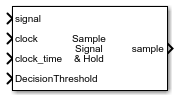# Signal Sampler

Sample incoming signal at the edge of incoming clock

• Library:
• Mixed-Signal Blockset / Utilities

•## Description

The Signal Sampler block estimates the value of an incoming fixed step discrete sampled signal at a specific time that is typically between two sample times. The block obtains this estimate using an accurate input value for the time and linear interpolation for the signal.

The Signal Sampler block calculates the time interval from the last sample to the exact time at which the clock transition occurred as the modular residue with respect to the sample interval. The block uses linear interpolation to calculate the new output sample value.

## Ports

### Input

expand all

Fixed step discrete input signal to be sampled, specified as a scalar.

Data Types: `single` | `double` | `int8` | `int16` | `int32` | `uint8` | `uint16` | `uint32` | `fixed point`

Saturated but sampled clock waveform, specified as a scalar. The input at the clock port, along with the input at the clock_time port, is used to define the time at which to sample the input signal.

Data Types: `double`

Exact time of the clock transition, specified as a scalar. The input at the clock_time port, along with the input at the clock port, is used to define the time at which to sample the input signal.

The voltage at which latched output switches polarity, specified as a scalar.

Data Types: `double`

### Output

expand all

Sample output value at the clock edge, returned as a scalar.

The output has two modes:

• Continuous — the output is exactly the value of the input signal at the time of the clock edge.

• Latched — the output is binary, with values `-1` or `1`.

Data Types: `single` | `double` | `int8` | `int16` | `int32` | `int64` | `uint8` | `uint16` | `uint32` | `uint64` | `Boolean` | `fixed point`

## Parameters

expand all

Main

Determine when the input signal is sampled, either the rising or falling edge of the clock signal.

#### Programmatic Use

 Block parameter: `ClockEdge` Type: character vector Values: `Rising`| `Falling` Default: `Rising`

Determine the output sample mode, either `Continuous` or `Latched`.

In `Continuous` mode, the output is exactly the value of the input signal at the time of the clock edge.

In `Latched` mode, the output is binary, with values `-1` or `1`.

#### Programmatic Use

 Block parameter: `OutputMode` Type: character vector Values: `Continuous`| `Latched` Default: `Continuous`

Select the simulation mode. This choice affects the simulation performance.

Simulating the model using the `Code generation` method requires additional startup time, but the subsequent simulations run faster. Simulating the model using the `Interpreted execution` method may reduce the startup time, but the subsequent simulations run slower. For more information, see Simulation Modes.

#### Programmatic Use

 Block parameter: `SimulateUsing` Type: character vector Values: `Code generation`| ```Interpreted execution``` Default: `Code generation`
Latched Output

Select to turn on the decision threshold port. When turned on, the voltage at the DecisionThreshold port is used to decide when to switch polarity for latched output. By default, this option is selected.

Decision threshold is supported as an AMI parameter.

#### Programmatic Use

 Block parameter: `DecisionThresholdPort` Type: character vector Values: on| off Default: on

The voltage at which latched output switches polarity, specified as a scalar. Use this parameter to define the decision threshold for latched output in the block itself.

Decision threshold is supported as an AMI parameter.

#### Dependencies

To enable this parameter, deselect the DecisionThresholdPort parameter.

#### Programmatic Use

 Block parameter: `DecisionThreshold` Type: character vector Values: scalar Default: `0`

The voltage above or below the decision threshold needed to assure that the latched output switches to the correct polarity. This parameter is used to model the metastable region of the latch. The behavior is modeled as the worst case, in which the latched output remains unchanged unless the sampled voltage is outside the metastable region. If the data sample voltage lies within the region (±Latch sensitivity), there is a 50% probability of bit error.

Latch sensitivity is supported as an AMI parameter.

#### Programmatic Use

 Block parameter: `Sensitivity` Type: character vector Values: scalar Default: `0`

Time of a single symbol duration, specified as a real positive scalar in seconds.

#### Programmatic Use

 Block parameter: `SymbolTime` Type: character vector Values: real positive scalar Default: `100e-12`

Data Types: `double`

Uniform time step of the waveform, specified as a real positive scalar in seconds.

#### Programmatic Use

 Block parameter: `SampleInterval` Type: character vector Values: real positive scalar Default: `6.25e-12`

Data Types: `double`

Number of logic levels in the modulation scheme:

• Select `2` if the modulation scheme is NRZ(non-return to zero).

• Select `3` if the modulation scheme PAM3 (pulse amplitude modulation level 3).

• Select `4` if the modulation scheme PAM4 (pulse amplitude modulation level 4).

#### Programmatic Use

 Block parameter: `Modulation` Type: character vector Values: `2`.| `3` | `4` Default: `2`

Data Types: `char`

Type of input waveform, either a sample by sample signal or an impulse response signal.

#### Programmatic Use

 Block parameter: `WaveType` Type: character vector Values: `Sample`.| `Impulse` Default: `Sample`

## Version History

Introduced in R2022a